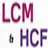# LCM & HCFQuantitative Aptitude Questions and Answers section on “LCM & HCF” with solution and explanation for competitive examinations such as CAT, MBA, SSC, Bank PO, Bank Clerical and other examinations.

# If the students of a class can be grouped exactly into 6 or 8 or 10, then the minimum number of students in the class must be :

If the students of a class can be grouped exactly into 6 or 8 or 10, then the minimum number of students in the class must be : [A]60 [B]120 [C]180 [D]240 Show Answer 120 Because the required number of students = LCM of 6, 8, 10 = 120. Hence option [B] is correct answer.

# The smallest number, which when divided by 12 and 16 leaves remaining 5 and 9 respectively, is :

The smallest number, which when divided by 12 and 16 leaves remaining 5 and 9 respectively, is : [A]29 [B]39 [C]41 [D]55 Show Answer 41 Here because, 12 – 5 = 7, 16 – 9 = 7 Since required number = LCM of 12 and 16 – 7 = 48 – 7 = 41. Hence ..

# Which is the least number which when double will be exactly divisible by 12, 18, 21 and 30?

Which is the least number which when double will be exactly divisible by 12, 18, 21 and 30? [A]196 [B]630 [C]1260 [D]2520 Show Answer 630 The LCM of 12, 18, 21, 30 is 1260. ∴ The required Number = Hence option [B] is correct answer.

# The least multiple of 13, which on dividing by 4, 5, 6, 7 and 8 leaves remainder 2 in each case is :

The least multiple of 13, which on dividing by 4, 5, 6, 7 and 8 leaves remainder 2 in each case is : [A]840 [B]842 [C]2520 [D]2522 Show Answer 2522 LCM of 4, 5, 6, 7 and 8 = 840. Let require number be 840 K + 2 which is multiple of 13. Least value ..

# The least number which when divided by 4, 5 and 6 leaves remainder 1, 2 and 3 respectively, is :

The least number which when divided by 4, 5 and 6 leaves remainder 1, 2 and 3 respectively, is : [A]57 [B]59 [C]61 [D]63 Show Answer 57 Here 4 – 1 = 3, 5 – 2 = 3, 6 – 3 = 3 ∴ The required Number = LCM of (4, 5, 6) – 3 ..